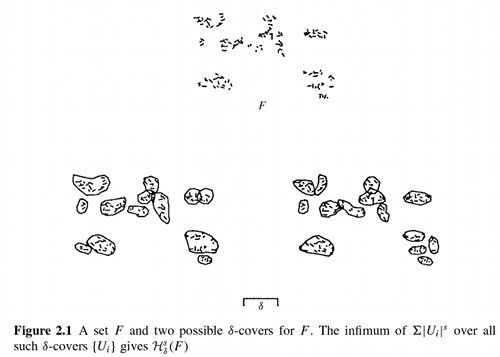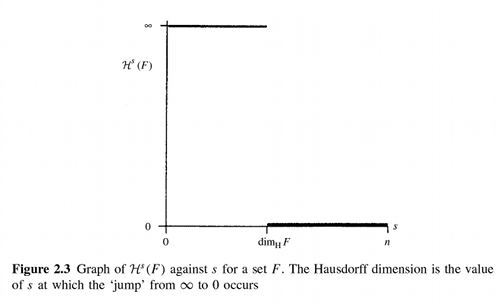# Fractal Dimension

Posted in: Math

After the previous post I thought it would be interesting to compare the topological dimension to the fractal dimension as it is presented in Kenneth Falconer’s Fractal Geometry. A fractal is commonly defined as an object which has a Hausdorff-Besicovitch dimension strictly greater than its topological dimension.

Quoting from the book:

## Hausdorff measure

Recall that if $$U$$ is any non-empty subset of n-dimensional Euclidean space, $$R^n$$, the diameter of $$U$$ is defined as $$|U| = sup\{|x-y|: x,\ y\ \epsilon\ U\}$$, i.e. the greatest distance apart of any pair of points in $$U$$. If $$\{U_i\}$$ is a countable (or finite) collection of sets of diameter at most $$\delta$$ that cover $$F$$, i.e. $$F \subset \bigcup_{i=1}^\infty\ U_i$$ with $$0 \leq |U_i| \leq \delta$$ for each $$i$$, we say that $$\{U_i\}$$ is a $$\delta$$-cover of $$F$$.

Suppose that $$F$$ is a subset of $$R^n$$ and $$s$$ is a non-negative number. For any $$\delta \gt 0$$ we define

 $$H_{\delta}^s(F) = inf\left(\sum_{i=0}^\infty U_i ^s \right)$$: $$\{U_i\}$$ is a $$\delta$$-cover of $$F$$

Thus we look at all covers of $$F$$ by sets of diameter at most $$\delta$$ and seek to minimize the sum of the $$s$$th powers of the diameters. As $$\delta$$ decreases, the class of permissible covers of $$F$$ is reduced. Therefore, the infimum $$H_{\delta}^s(F)$$ increases, and so approaches a limit as $$delta \to 0$$. We write

$$H^s(F) = \lim_{\delta \to 0} H_{\delta}^s(F)$$

This limit exists for any suybset $$F$$ of $$R^n$$, though the limiting value can be (and usually is) $$0$$ or $$\infty$$. We call $$H^s(F)$$ the s-dimensional Hausdorff measure of F.

(…)## Hausdorff dimension

If $$t \gt s$$ and $$\{U_i\}$$ is a $$\delta$$-cover of $$F$$ we have

 $$\sum_i U_i ^t \leq \sum_i U_i ^{t-s} U_i ^s \leq \delta^{t-s} \sum_i U_i ^s$$

so, taking infima, $$H_{\delta}^t(F) \leq \delta^{t-s} H_{\delta}^s(F)$$. Letting $$\delta \to 0$$ we see that if $$H^s(F) \lt \infty$$ then $$H^t(F) = 0$$ for $$t \gt s$$. Thus a graph of $$H^s(F)$$ against $$s$$ shows that there is a critical value of $$s$$ at which $$H^s(F)$$ ‘jumps’ from $$\infty$$ to $$0$$. This critical value is called the Hausdorff dimension of $$F$$, and written $$dim_H F$$; it is defined for any set $$F \subset R^n$$. (Note that some authors refer to Hausdorff dimension as Hausdorff-Besicovitch dimension). Formally

$$dim_H F = inf\{s \geq 0\ :\ H^s(F) = 0\} = sup\{s\ :\ H^s(F) = \infty\}$$